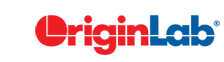OriginPro
Searching...
No Matches
originpro.analysis.LinearFit Class Reference

## Public Member Functions

def __init__ (self)

def __del__ (self)

def set_data (self, wks, x, y, err='')

def fix_slope (self, val)

def fix_intercept (self, val)

def result (self)

def report (self, band=0)

## Detailed Description

```class for performing Linear Fitting with Origin's internal fitting engine
```

## ◆ fix_intercept()

 def originpro.analysis.LinearFit.fix_intercept ( self, val )
```fix intercept to a value or to turn off the fixing
```

## ◆ fix_slope()

 def originpro.analysis.LinearFit.fix_slope ( self, val )
```fix slope to a value or to turn off the fixing
```

## ◆ report()

 def originpro.analysis.LinearFit.report ( self, band = `0` )
```perform the fitting and generate the report. You need to end the fitting by either calling result or report

Parameters:
band (int): confidence and prediction bands. 0=none,1=confidence,2=prediction,3=both

Returns:
(tuple): range strings of the report sheet and the fitted curves

Examples:
lr = op.LinearFit()
lr.set_data(wks, 1, 2)
r, c = lr.report(1)
wReport=op.find_sheet('w', r)
wCurves=op.find_sheet('w', c)
```

## ◆ result()

 def originpro.analysis.LinearFit.result ( self )
```perform the fitting and return the parameters. You need to end the fitting by either calling result or report

Return:
(dict) fitting parameters and statistics from the fit

Examples:
lr = op.LinearFit()
lr.set_data(wks, 1, 2)
rr = lr.result()
b       =rr['Parameters']['Slope']['Value']
b_err   =rr['Parameters']['Slope']['Error']```

## ◆ set_data()

 def originpro.analysis.LinearFit.set_data ( self, wks, x, y, err = `''` )
```set the XY data with optional error bar column
```

The documentation for this class was generated from the following file:
• src/originpro/analysis.py